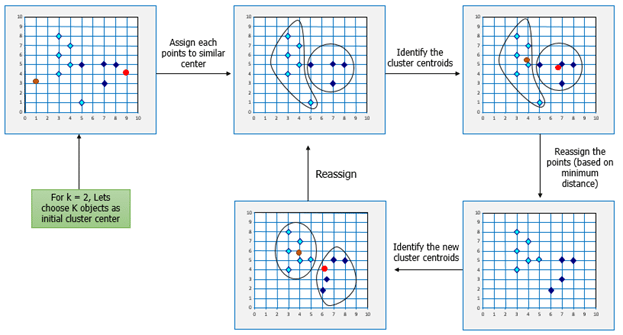I am basically looking for Python implementation of k-means algorithm with examples to cluster and cache my database of coordinates. Any help would be appreciated.Sep 9 in Python 47 views

1 answer to this question.

What is K-means Clustering?

K-means (Macqueen, 1967) is one of the simplest unsupervised learning algorithms that solve the well-known clustering problem. K-means clustering is a method of vector quantization, originally from signal processing, that is popular for cluster analysis in data mining.

K-means Clustering – Example 1:

A pizza chain wants to open its delivery centers across a city. What do you think would be the possible challenges?

• They need to analyze the areas from where the pizza is being ordered frequently.
• They need to understand how many pizza stores has to be opened to cover delivery in the area.
• They need to figure out the locations for the pizza stores within all these areas in order to keep the distance between the store and delivery points minimum.

Resolving these challenges includes a lot of analysis and mathematics. We would now learn about how clustering can provide a meaningful and easy method of sorting out such real-life challenges. Before that let’s see what clustering is.

K-means Clustering Method:

If k is given, the K-means algorithm can be executed in the following steps:

• Partition of objects into k non-empty subsets
• Identifying the cluster centroids (mean point) of the current partition.
• Assigning each point to a specific cluster
• Compute the distances from each point and allot points to the cluster where the distance from the centroid is minimum.
• After re-allotting the points, find the centroid of the new cluster formed.

The step by step process:answered Sep 9 by
• 2,960 points

Explain OOPs concept in python with programatic examples

OOPS concept in Python is huge. I ...READ MORE

How can I deal with python eggs for multiple platforms in one location?

Try virtualenv : http://pypi.python.org/pypi/virtualenv This helps you create isolated ...READ MORE

How can I replace values with 'none' in a dataframe using pandas

Actually in later versions of pandas this ...READ MORE

How can I plot a k-dsitance graph using python?

Hi there, instead of sklearn you could ...READ MORE

how can i randomly select items from a list?

if you google it you can find. ...READ MORE

+1 vote

how can i count the items in a list?

Syntax :            list. count(value) Code: colors = ['red', 'green', ...READ MORE

how do i use the enumerate function inside a list?

can you give an example using a ...READ MORE

Lowercase in Python

You can simply the built-in function in ...READ MORE